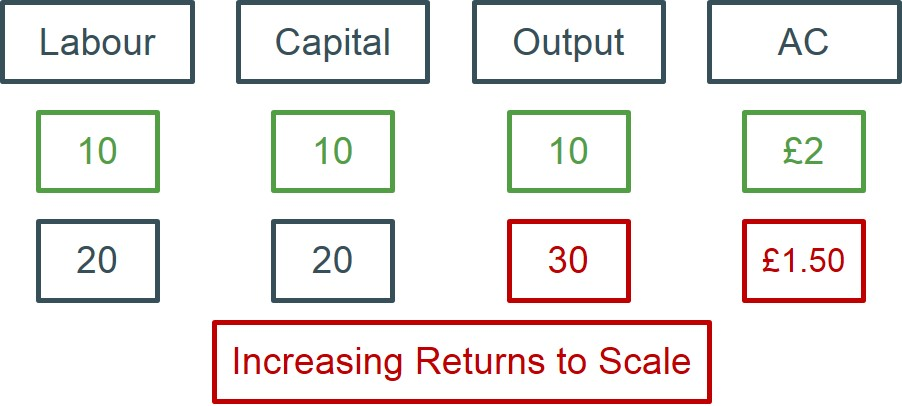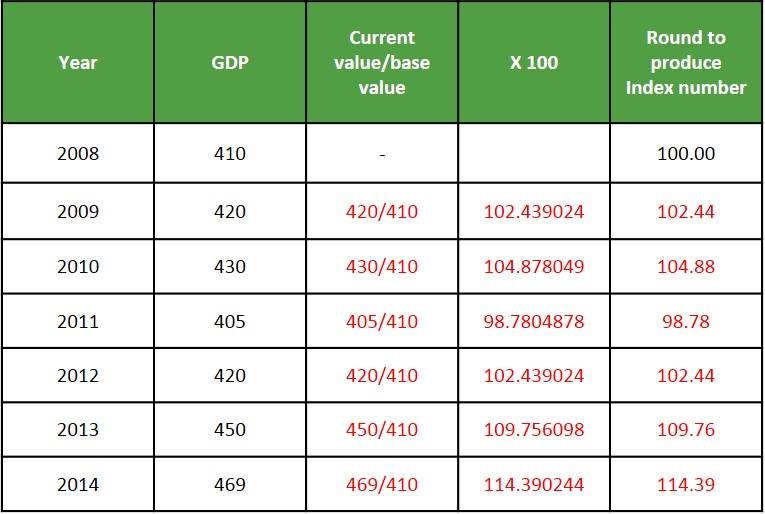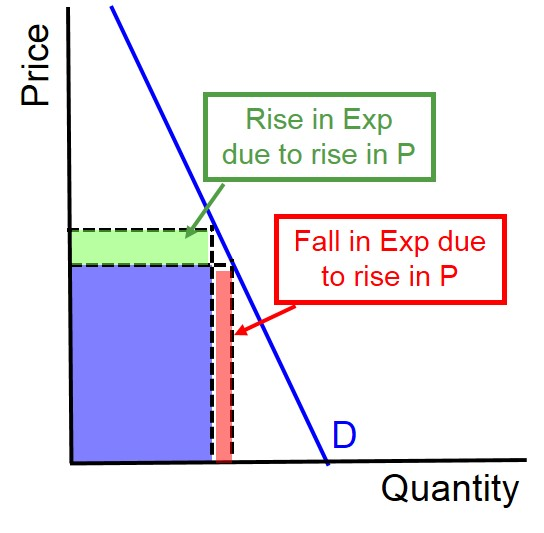The EzyEducation website uses cookies to help ensure we give you the best experience.
If you continue without changing your settings, we assume that you are happy to receive all cookies on the EzyEducation website.

Continue

# Economic Terms

### Income tax

A direct tax imposed on income from employment and investments. assessable earnings are taxed at either the basic (20%), higher (40%) or additional rate (45%).

### Increasing Returns to Scale

When a firm increases all the factors of production by a factor and output increases by more than that factor. As a result the average cost for the firm will be falling as output exceeds inputs.

Below is an illustration of how a business would achieve increasing returns to scale. Assuming this firm only uses capital and labour as its inputs. A doubling of the capital and labour input leads to greater than doubling of output as well. Because the average cost is calculated by the total costs/output, if the costs are increasing slower than output, average costs fall over time.### Incumbent Firm

Describes a firm that is already in position in the market. In a contestable market incumbent firms are unable to enjoy abnormal profits due to hit-and-run entry.

### Index numbers

A way of simplifying the measurement of averages (e.g. inflation and stock markets) and comparing different data series by establishing a base of 100.

Index numbers are calculated by selecting a base year and then dividing each of the values by that base year value and seeing the proportionate change relative to the base year rather than the percentage change. It is important to remember that index numbers DO NOT show the percentage change in a variable over time. The main benefit of index numbers is they allow cross-variable comparisons with variables that use different measurements. The table shows how the index numbers for GDP are calculated using the method mentioned above.### Indirect tax

Taxes that are imposed on transactions. e.g. VAT, fuel duty, insurance premium tax.

### Indirect taxation

Taxes that are imposed on transactions. e.g. VAT, fuel duty, insurance premium tax.

### Indirect taxes

Taxes that are imposed on transactions. e.g. VAT, fuel duty, insurance premium tax.

### Individual demand

The demand for a good/service from an individual consumer or firm.

### Indivisibilities

This is a technical economy of scale. Many economies of scale can only be captured by using large items of equipment that must be continuously used to full capacity. This means that smaller suppliers can’t access the same economies of scale if the equipment isn’t available on a smaller scale.

### Inelastic demand

When the percentage change in demand is less than the percentage change in price. In this case the PED elasticity value will lie between 0 and -1.

To identify the shape of an inelastic linear demand curve we do not focus on the gradient of the curve as this does not actually determine elasticity. Crucially, it is the position of the demand curve that determines elasticity. This is because all linear demand curves contain portions on the curve which can be classed as elastic, inelastic and unit elastic. Any demand curve shown (like the one below) is just a section of a much larger demand curve that extends beyond the axis.So effectively we can see that the midpoint of the demand curve is when unitary elasticity is achieved. The bottom half of the curve is inelastic, because if the price rises - at any point below the midpoint - expenditure increases despite a quantity fall. The top half of the curve is elastic, because if the prices rises - at any point above the midpoint - expenditure decreases due to a large quantity fall.

Therefore, the easiest way to determine an inelastic demand curve is to extend the section of the demand curve drawn and identify which axis it intersects. If the demand curve intersects the x axis we can judge it as being an inelastic demand curve as we must be focusing on the bottom of the demand curve. An example of an inelastic demand curve is shown below.This diagram highlights the changes in expenditure for a producer that occurs when there is a small price rise in a market with inelastic demand. When a demand curve is relatively inelastic, it means that consumers are price insensitive to changes. In this instance, a price rise leads to a small fall in demand as consumers refuse to switch to alternative cheaper substitutes

Display #